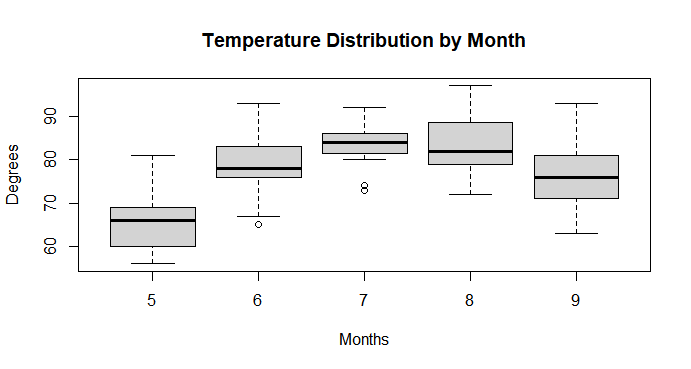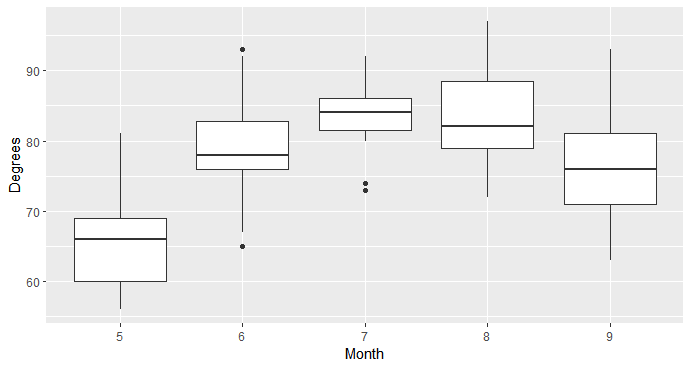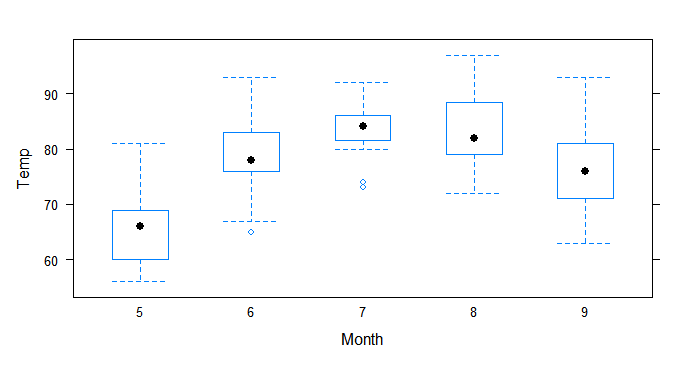Open in App
Not now

# Draw Multiple Boxplots in One Graph using R

• Difficulty Level : Medium
• Last Updated : 11 Jul, 2022

In this article, we are learning about Drawing Multiple BoxPlots in One Graph in the R programming language.

## Method 1: Multiple BoxPlot in One Graph Using Base R

In this method to plot multiple boxplots in one graph, the user needs a box and whisker plot in base R can be plotted with the boxplot function.

Syntax: boxplot(x,data,notch,varwidth,names,main)

Parameters:

•  x: This parameter sets as a vector or a formula.
• data: This parameter sets the dataframe.
• notch: This parameter is the label for the horizontal axis.
• varwidth: This parameter is a logical value. Set as true to draw the box width proportionate to the sample size.
• main: This parameter is the title of the chart.
• names: These parameters are the group labels that will be shown under each boxplot.

Example:

To illustrate how to create boxplots in base R, we’ll work with the built-in air quality dataset in R :

## R

 `summary``(airquality) ``# Summary of Dataset ` ` `  `boxplot``(Temp ~ Month,data = airquality,  ` `        ``main = ``"Temperature Distribution by Month"``, ` `        ``xlab = ``"Months"``,ylab = ``"Degrees"``) `

Output:

The above codes generate the following chart that displays multiple BoxPlots for each Month.## Method 2: Multiple BoxPlot in One Graph Using ggplot2

The ggplot2 is a plotting package that provides helpful commands to create complex plots from data in a data frame. ggplot2 refers to the name of the package itself. When using the package we use the function ggplot() to generate the plots, and so references to using the function will be referred to as ggplot() and the package as a whole as ggplot2.

Syntax: ggplot(data = NULL, mapping = aes(), …, environment = parent.frame())

Parameters:

• data: Default dataset to use for plot. If not already a data.frame, will be converted to one by fortify().
• mapping: Default list of aesthetic mappings to use for plot.
• … : Other arguments passed on to methods.
• environment: DEPRECATED. Used prior to tidy evaluation.

Example:

In this example, we will use the built-in air quality dataset to generate the following chart that displays one boxplot for each month using the ggplot() function with the grom_boxplot() function from the ggplot2 package of the R language.

## R

 `library``(ggplot2) ``# Load ggplot2 ` ` `  `ggplot``(data = airquality, ``aes``(x=``as.character``(Month), y=Temp)) +  ` `    ``geom_boxplot``() + ` `    ``labs``( x=``"Month"``, y=``"Degrees"``)`

Output:

The above codes generate the following chart that displays multiple BoxPlots for each Month.## Method 3: Multiple BoxPlot in One Graph Using lattice

The function bwplot() makes box-and-whisker plots for numerical variables. It comes from the lattice package for statistical graphics, which is pre-installed with every distribution of R.

Syntax : bwplot(x, by, data, xlab = “”,  ylab =””,main = “”, space = 0.25, …)

Parameters:

• x: name of the variable to be plotted.
• by: the name of the factor variable to be used to subdivide the data.
• data: Default dataset to use for plot.
• xlab: a title for the x-axis, by default none is provided.
• ylab: a title for the y-axis.
• space: the space between the individual boxplots, by default this is 0.25 x-axis units.
• … : further arguments to be passed to methods. For example, the size of the axis titles by setting cex.lab, and the size of the plot title by setting cex.main.

In this example. we will use the built-in airquality dataset to generate the chart with the bwplot() function from the lattice package of the R language.

## R

 `library``(``"lattice"``) ``# Load lattice package ` ` `  `bwplot``(Temp ~ ``as.character``(Month), data = airquality,xlab = ``"Month"``)`

Output:

The above codes generate the following chart that displays multiple BoxPlots for each Month.My Personal Notes arrow_drop_up
Related Articles